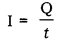# (a) How is the direction of electric current related to the direction of flow of electrons in a wire?

(a) How is the direction of electric current related to the direction of flow of electrons in a wire?
(b) Calculate the current in a circuit of 500C of charge passes through it in 10 minutes.

(a) The direction of the electric current flowing through a wire is opposite to the direction of flow of electrons through the wire.

The direction of flow of current in a circuit is taken by convention, as the direction of flow of positive charges, i.e., from the positive terminal to the negative terminal of a battery, through the external circuit. Since, the direction of flow of electrons (negative charges) in a circuit is opposite to the direction of flow of positive charges, the direction of flow of electric current is opposite to the direction of flow of positive charges, the direction of flow of electric current is opposite to the direction of flow of electrons in a circuit/wire.

(b) The current (l) flowing through a circuit is given by the amount of total charges (Q) passing in a unit time (t). It is given by:It is given that: Q = Total charge = 500 C ; t = 10 min. = 600 sec.Hence, 0.83A current flows through the circuit.